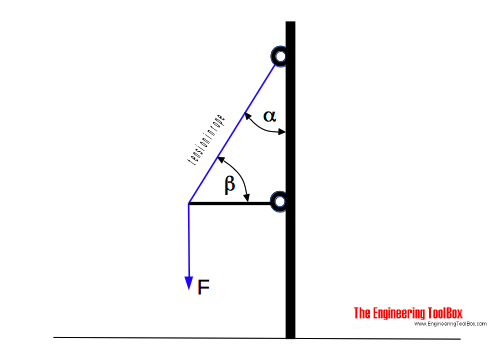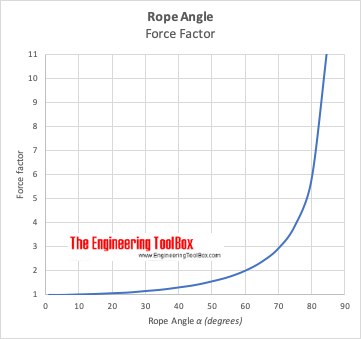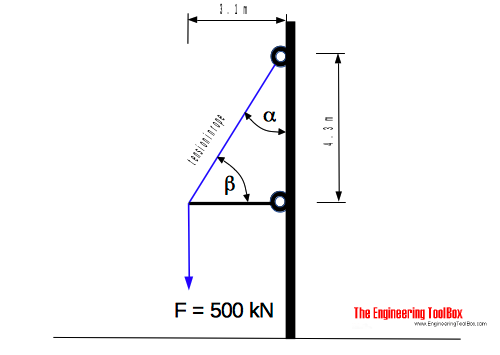Engineering ToolBox - Resources, Tools and Basic Information for Engineering and Design of Technical Applications!

# Forces and Tensions in Ropes due to Angle

## Reduced load capacities in ropes, cables or lines - due to acting angle.The increased force or tension in a rope or cable due to angle:

Rope Angle with Load (degrees)Increased Force or Tension Factor
- θ -
- α -
- β -
0 90 1.00
5 85 1.00
10 80 1.02
15 75 1.04
20 70 1.07
25 65 1.10
30 60 1.16
35 55 1.22
40 50 1.31
45 45 1.41
50 40 1.56
55 35 1.74
60 30 2.00
65 25 2.37
70 20 2.92
75 15 3.86
80 10 5.76
85 5 11.5

As we can see from the table above - with

α angle = 60 degrees

and

β angle = 30 degrees

the force or tension F in the rope is doubled.The force acting in the rope can be calculated as

Frope = θ F                 (1)

where

Frope = force acting in the rope (N, lb)

θ = increased force or tension factor from the table above

The force acting in the horizontal beam can be calculated as

Fbeam = (Frope2 + F2)1/2                           (2)

The angle α can be calculated as

α = tan-1(h / d)                 (3)

where

α = angle (degrees)

h = vertical distance between horizontal beam and rope (m, ft)

d = length of horizontal beam (m, ft)

The angle β can be calculated as

β = tan-1(d / h)                 (4)

where

β = angle (degrees)

### Example - Increased Force in a Rope due to AngleThe maximum force in the rope in the figure above can be estimated by firs calculate the angles:

α = tan-1(3.1 / 4.3)

= 35.8 o

β = tan-1(4.3 / 3.1)

= 54.2 o

From the table above the tension factor is approximately 1.22 and the force in the rope can be calculated as

Frope = (500 kN) 1.22

= 610 kN

height (m, ft)

depth (m, ft)

## Related Topics

• Mechanics - Forces, acceleration, displacement, vectors, motion, momentum, energy of objects and more.
• Statics - Loads - forces and torque, beams and columns.

## Engineering ToolBox - SketchUp Extension - Online 3D modeling!

Add standard and customized parametric components - like flange beams, lumbers, piping, stairs and more - to your Sketchup model with the Engineering ToolBox - SketchUp Extension - enabled for use with the amazing, fun and free SketchUp Make and SketchUp Pro .Add the Engineering ToolBox extension to your SketchUp from the SketchUp Pro Sketchup Extension Warehouse!

Translate

## Privacy

We don't collect information from our users. Only emails and answers are saved in our archive. Cookies are only used in the browser to improve user experience.

Some of our calculators and applications let you save application data to your local computer. These applications will - due to browser restrictions - send data between your browser and our server. We don't save this data.

## Citation

• Engineering ToolBox, (2009). Forces and Tensions in Ropes due to Angle. [online] Available at: https://www.engineeringtoolbox.com/rope-angle-tension-increase-d_1507.html [Accessed Day Mo. Year].

Modify access date.

. .

#### Scientific Online Calculator3 30